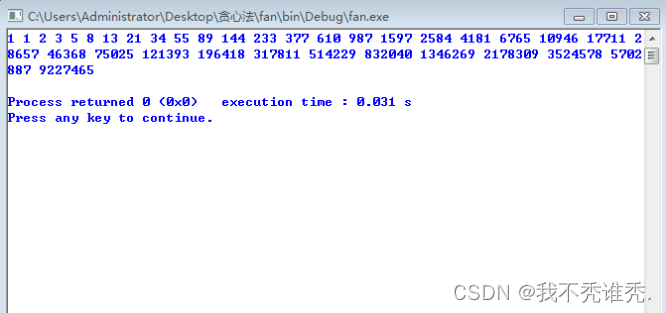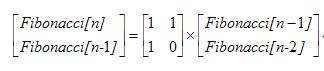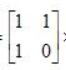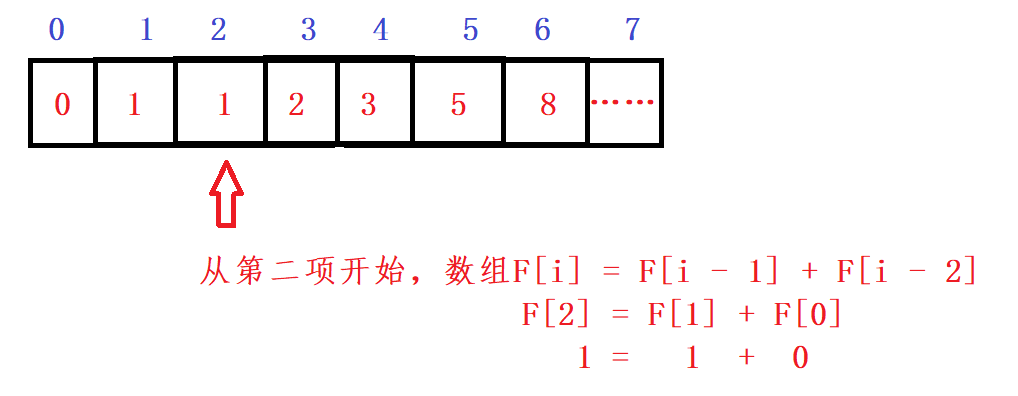• 斐波那契数列(动态规划法)
2022-04-29 17:25:57

写一个函数，输入 n ，求斐波那契（Fibonacci）数列的第 n 项（即 F(N)）。斐波那契数列的定义如下：

F(0) = 0, F(1) = 1

F(N) = F(N - 1) + F(N - 2), 其中 N > 1.

斐波那契数列由 0 和 1 开始，之后的斐波那契数就是由之前的两数相加而得出。

答案需要取模 1e9+7（1000000007），如计算初始结果为：1000000008，请返回 1。

示例 1：

输入：n = 2

输出：1

示例 2：

输入：n = 5

输出：5

提示：

   0 <= n <= 100

解题思路：动态规划法

因为斐波那契数列的性质：f(n+1)=f(n)+f(n−1)

所以可以从f(0)=0，f(1)=1开始往后算，由于用传统的递归函数会产生大量的重复计算， 所以可以迭代，令a=f(0)，b=f(1)，sum=a+b，然后让a=b，b=sum，sum=a+b，继续更替a和b。因为随着n的增大，f(n)会打过int的范围，假设int是32位，只能存储2^(32-1)为，所以为了防止出现结果超出int范围的情况，需要将结果%1000000007(这个是十位数中最小的质数)

算法流程：

1.定义a=f(0)=0，b=f(1)=1，sum=0

2.循环 n 次

sum=(a+b)%1000000007;

a=b;

b=sum;

3.返回 a

代码：

class Solution {

public:

int fib(int n) {

// 第一步

int a=0,b=1,sum=0;

//第二步

for(int i=0;i<n;i++) {

sum=(a+b)%1000000007;

a=b;

b=sum;

}

// 第三步

return a;

}

};算法
更多相关内容
• 课程的随堂作业，C语言的，dev就能运行，萌新代码，勿喷，仅仅帮助不想写作业的朋友方便一下，反正老师也不会仔细检查的C语言
• 动态规划法求解斐波那契数列第n项，要求两种算法思路（一种空间复杂度为O(n)，一种空间复杂度小于O(n)）。 用到的思想—动态规划法 博主用到的环境：Win7, CodeBlocks等。 一、代码 #include <iostream> ...

算法经典题型23

## 动态规划法求解斐波那契数列第n项，要求用两种算法思路（一种空间复杂度为O(n)，一种空间复杂度小于O(n)）。

用到的思想—动态规划法
博主用到的环境：Win7, CodeBlocks等。

# 一、代码

#include <iostream>
using namespace std;

long fabi1(int n,long *s);

int main()
{
int i;
long s;
for(i=0;i<1024;i++)
{
s[i]=0;
}
for(i=0;i<35;i++)
{
cout <<fabi1(i+1,s)<<" ";
}
cout <<endl;
return 0;
}

long fabi1(int n,long *s)
{
if(n <=1 )
{
return n;
}
s[n-1]=fabi1(n-1,s);
return s[n-1]+s[n-2];
}


# 二、测试# 总结

谢谢宝宝们的阅读，有问题的话评论@我，没问题的话点个赞再走哦~展开全文c++ 算法 后端 编程语言
• O(logN)求斐波那契数列第N项动态规划、矩阵分治

# logN求Fibonacci数列第N项

• 斐波那契数列通项公式 F ( i ) = F ( i − 1 ) + F ( i − 2 ) F(i)=F(i-1)+F(i-2)
• 以下介绍两种复杂度为 O ( l o g N ) O(logN) 的算法
• 两种方法思想类似

# 1. 动态规划

• 当我们尝试将 F ( i − 1 ) F(i-1) F ( i − 2 ) F(i-2) 进行继续拆解时（始终保持两项），发现两项的系数始终是斐波那契数列中的某一相邻两项
• 因此，F(i)一定可以可以由4个项组成
• F ( i ) = F ( i − a ) F ( i − x ) + F ( i − b ) F ( i − ( x − 1 ) ) F(i)=F(i-a)F(i-x)+F(i-b)F(i-(x-1))
• a = 0 a=0 时则为 F ( i ) = F ( 2 ) F ( i − 1 ) + F ( 1 ) F ( i − 2 ) F(i)=F(2)F(i-1)+F(1)F(i-2)
• 且a，b，x具有一定关系

## 思路

a，b，x有多种可能
此时需要算4个小项才能合并到1个大项
若这4个项中有相同的项，则可以简化计算
此外，越大的项，计算的复杂度越大，反之越小

# 因此我们可以想到取 i / 2 i/2 附近的项

• 通过递推得（也可以用较小的 i i 找找规律）
• i i 为奇数时， F ( i ) = F 2 ( i / 2 + 1 ) + F 2 ( i / 2 ) F(i)=F^2(i/2+1)+F^2(i/2)
• i i 为偶数时， F ( i ) = F ( i / 2 + 1 ) ∗ F ( i / 2 ) + F ( i / 2 ) ∗ F ( i / 2 − 1 ) F(i)=F(i/2+1)*F(i/2)+F(i/2)*F(i/2-1)

# 即我们将一个递推项分成2或3项分别计算后再合并

• 可以分析复杂度为 O ( l o g N ) O(logN)
• ：在实际程序实现中，不能直接return F(i/2+1)*F(i/2)+F(i/2)*F(i/2-1)，这样会造成计算冗余
• 应该先保存下结果，计算后return

# DP代码

int Fibonacci(int x) // logN
{
if (x == 1 || x == 2) return 1;
if (x % 2 != 0)
{
int F1 = Fibonacci(x / 2 + 1), F2 = Fibonacci(x / 2);
return F1 * F1 + F2 * F2;
}
else
{
int F1 = Fibonacci(x / 2 + 1), F2 = Fibonacci(x / 2), F3 = Fibonacci(x / 2 - 1);
return F1 * F2 + F2 * F3;
}
}


# 2. 矩阵分治

• 结合矩阵的知识，我们可以知道斐波那契数列符合
•• 于是随着等号右边中的斐波那契项的项数 i i 降低，该矩阵就不断左乘

# 因此可以基于矩阵结合律计算若干项矩阵乘积，再左乘 F ( 1 ) a n d F ( 2 ) F(1) and F(2)

• 设该矩阵为A
• A n = A n / 2 ∗ A n / 2 A^n=A^{n/2}*A^{n/2}
• 其中 A n / 2 A^{n/2} 只需计算一项
• 依次类推

# 代码

int Matrix(int x)
{
int a = { { 1, 1 }, { 0, 1 } };
if (x == 1) return a;
int b = Matrix(x / 2);
if (x % 2 == 0) return b * b; // 省略矩阵乘法
return b * b * a;
}

展开全文算法 分治算法
• 斐波那契数列的高效求法-动态规划，内含两份代码，一份是递归求解，另一个是动态规划
• 动态规划问题一般从以下四个角度考虑： 1. 状态定义 2. 状态间的转移方程定义 3. 状态的初始化 4. 返回的结果 状态定义的要求：定义的状态一定要形成递推关系。 一句话概括：三特点四要素本质 适用场景：最大值/...

# 一、基本概念

动规是非递归的一种代码可以保存下来一些过程的解
1. 把原来的为标题分解成几个相似的子问题
2. 所有的子问题都只需要解决一次
3. 储存子问题的解

动规本质：是对问题状态的定义和状态方程的定义（状态以及状态之间的递归关系）

动态规划问题一般从以下四个角度考虑：
1. 状态定义
2. 状态间的转移方程定义
3. 状态的初始化
4. 返回的结果

状态定义的要求：定义的状态一定要形成递推关系

一句话概括：三特点四要素本质

适用场景：最大值/最小值。可不可行。是不是，方案个数。

递归的思想还是要从实际问题切入来理解，

# 二、斐波那契数列

这道题是剑指Offer的一道题，非常经典

描述

大家都知道斐波那契数列，现在要求输入一个整数n，请你输出斐波那契数列的第n项（从0开始，第0项为0，第1项是1）。
n ≤ 39 n ≤ 39 n\leq 39n≤39

斐波那契数列

## 2.1 递归解法

首先，递归解法很容易理解，但是递归对n有很大的要求，太大会导致栈帧溢出，究其原因是每次返回的都是两个函数的递归，随着n的增大造成指数级增长。

class Solution {
public:
int Fibonacci(int n) {
if(n == 0)
return 0;
if(n == 1 || n ==2)
return 1;
return Fibonacci(n - 1) + Fibonacci(n - 2);
}
};


这道题递归解法空间复杂度是O(2^(n-1))，时间复杂度O(N)，这道题使用动规如何解呢？

## 2.2 动规解法

我们列出四要素：

问题：
数列第n项的值

状态F(i)：
数列第i项的值

转移方程：
F ( i ) = F ( i − 1 ) + F ( i − 2 ) F(i) = F(i - 1) + F(i - 2)
初始状态至少有两项：
F(0) = 0
F(1) = 1
返回值
F(n)

我们可以用一个数组把中间某一项的值保存下来，后一项的值就等于前两项值之和

class Solution {
public:
int Fibonacci(int n) {
//时间复杂度O（n）空间复杂度O(n)
vector<int> F(n + 1, 0);    //定义数组保存每一次的状态值
//初始化：F = 0, F = 1
F = 0;
F = 1;
//状态方程求解F[i] = F[i - 1] + F[i - 2]
for(int i = 2; i <= n; ++i)
{
F[i] = F[i - 1] + F[i - 2];
}
return F[n];
};这道题时间复杂度O（n），空间复杂度O(n)，依然可以继续优化

## 2.3 迭代解法

class Solution {
public:
int Fibonacci(int n) {
//时间复杂度O(n),空间复杂度是O(1)
//fn1代表f(n - 1), fn2代表f(n - 2)
int fn1 = 1;
int fn2 = 0;
int fn;
if(n <= 1)
return n;
for(int i = 2; i <=n; ++i)
{
fn = fn1 + fn2;
fn2 = fn1;
fn1 = fn;
}
return fn;
}
};


通过迭代，这道题时间复杂度O(n),空间复杂度是O(1)

展开全文c++ 算法
• 写一个函数，输入 n求斐波那契Fibonacci数列 n 斐波那契数列的定义如下： F(0) = 0, F(1) = 1 F(N) = F(N - 1) + F(N - 2), 其中 N > 1. 来源：力扣（LeetCode） 链接：...leetcode
• 动态规划 用于求解最优化子问题的，往往是高效的而准确的。这背后的逻辑，其实就是程序设计...而其实分解的子问题，往往会有许多重复的子问题，程序进行减枝机制地优化，这是动态规划法斐波那契数列大学课堂上，...
• c++动态规划法求斐波那契数列 问题描述：斐波那契数列，又称黄金分割数列，指的是这样一个数列：0、1、1、2、3、5、8、13、21、34、……在数学上，斐波纳契数列以如下被以递归的方法定义：F（0）=0，F（1）=1，F（n...算法 c++
• 对于菲波那切数列都不陌生了，不啰嗦题目，直接切入正题。 常规解法，也是一反应： 递归法 public int fib(int n) { if(n==0 || n==1){ return n;...动态规划法 public int fib(int n) {数据结构 java
• 文章目录斐波那契数列解题思路：**动态规划解析：****空间复杂度优化：****循环余法：****复杂度分析：**代码： 解题思路： 斐波那契数列的定义是 f(n + 1) = f(n) + f(n - 1)，生成 n项的做法有以下几种： ...java 算法
• 动态规划法与分治方法   动态规划（Dynamic Programming）与分治方法相似，都是通过组合子问题的解来求解原问题。不同的是，分治方法通常将问题划分为互不相交的子问题，递归地求解子问题，再讲它们的解组合起来...
• 对于斐波那契数列，常规的递归求解方法很费时间，因为一些重叠子问题进行了多次计算。常规方法如下 public static int Fib1(int n) {//递归算法,当n很大时效率很低，时间复杂度很高，指数级别的！ if(n<=1) ...算法 leetcode
•算法 leetcode
• 这个数列3开始，每一都等于前两之和。 1、递归求解 刚学习C语言的递归的同学们一般都会想到递归求解 。 typedef unsigned int u_int; u_int fac(u_int n) { if(n==1||n==2) ret算法
• 斐波那契数列是最经典的动态规划算法应用实例之一。接下来，将从斐波那契数列入手，学习下基于动态规划求解问题。算法
• 求斐波那契数列第n项，利用递归思想，除了一、二位，每一位都是前两的和。递归函数的临界点就是等于1或n等于2，n大于2时便再次调用前n-1和n-2的值然后相加。 //斐波那契数列 public class RecursionDemo03 { ...java 算法
• 问题描述：写一个函数，输入 n求斐波那契Fibonacci数列 n （即 F(N)）。斐波那契数列的定义如下： F(0) = 0, F(1) = 1 F(N) = F(N - 1) + F(N - 2), 其中 N > 1. 斐波那契数列由 0 和 1 开始，之后...算法
• 一提到动态规划，大多数人都想到许许多多高端的名词，如什么状态转移方程什么的...不过别急，本人我掌握动态规划的历程带你理解动态规划，让你成功进入动态规划的领域。 在使用动态规划前，先了解为什么使用动态规...算法 leetcode
• 最近在看《算法导论》视频，就顺手实现了下斐波那契数列求算： 先上基本解法，几乎是小白闭着眼都能写的代码： 为了不产生指数级别的时间复杂度，只需要将中间过程中计算的斐波那契数列数值都记录下就可，改进代码...算法导论
• 动态规划法求斐波那契数列第n个数 动态规划法求斐波那契数列第n个数的改进 矩阵乘法角度:斐波那契数列的O(logn)解法 分治法思想 分治法的适用条件: 问题的规模缩小到一定程度就可以容易地解决； 问题可以...
• 斐波那契数列Fibonacci sequence），又称黄金分割数列、因数学家列昂纳多·斐波那契（Leonardoda Fibonacci）以兔子繁殖为例子而引入，故又称为“兔子数列”，指的是这样一个数列：1、1、2、3、5、8、13、21、34、...
• 斐波那契数列Fibonacci sequence），又称黄金分割数列、因数学家列昂纳多·斐波那契（Leonardoda Fibonacci）以兔子繁殖为例子而引入，故又称为“兔子数列”，指的是这样一个数列：1、1、2、3、5、8、13、21、34、...
• ## Python之求斐波那契数列前n项

万次阅读 多人点赞 2019-09-06 22:06:10
源码如下： # fibo.py def fibo(n): a, b = 0, 1 for i in range(n): print(a,end='\t') a,b = b, a + b ...print('斐波那契n项'.center(20,'-')) fibo(n) 运行结果： 关键代码也就两三行 ...
• 动态规划解决斐波那契数列 动态规划问题的三个特点： 1.把原来的问题分解成几个相似的子问题。 2.所有的子问题都只需要解决一次。 3.存储子问题的解。 动态规划问题从四个角度考虑： 1.状态定义 2.状态间的转移方程...算法
• 1、动态规划算法：  动态规划：通过把原问题分解为相对简单的子问题的方式求解复杂问题的方法。... 通常许多子问题非常相似，为此动态规划法试图仅仅解决每个子问题一次，从而减少计算量： 一旦
• 如果一个递归动态规划的具体实现可以分为两类：一类是自顶向下的备忘录方法，这种方法只需要原始的递归算法进行少量的改动，增加一个子问题解的记录，每当需要用到一个子问题的解时，首先查看这个记录，如果记录中...
• 大家都知道斐波那契数列，现在要求输入一个整数n，请你输出斐波那契数列第n项（从0开始，0项为0）。（n<=39） 提示：要用到动态规划，否则无法达成执行时间的限制 代码如下： import java.util.Scanner; ...java...